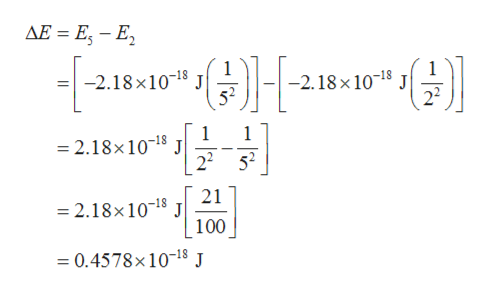# Recall Planck's constant equals 6.63 * 10-34 J*s and the speed of light is 3.00 * 108 m/s.Calculate the wavelength (in nm) of a photon emitted by a hydrogen atom when its electron drops from the n = 5 state to the n = 2 state.

Question

Recall Planck's constant equals 6.63 * 10-34 J*s and the speed of light is 3.00 * 10m/s.

Calculate the wavelength (in nm) of a photon emitted by a hydrogen atom when its electron drops from the n = 5 state to the n = 2 state.

check_circle

Step 1

The values are given as follows:

Step 2

The energy of the electron for the n quantum number can be expressed as follows:

Step 3

The difference between the electron’s energy from...help_outlineImage TranscriptioncloseAE E - E -2.18x1018 J 52 -2.18 x 10-18 J 22 1 =2.18x 1018 J 1 2252 21 J 100 2.18x 1018 =0.4578x 10-18 J fullscreen

### Want to see the full answer?

See Solution

#### Want to see this answer and more?

Solutions are written by subject experts who are available 24/7. Questions are typically answered within 1 hour.*

See Solution
*Response times may vary by subject and question.
Tagged in

### Chemistry